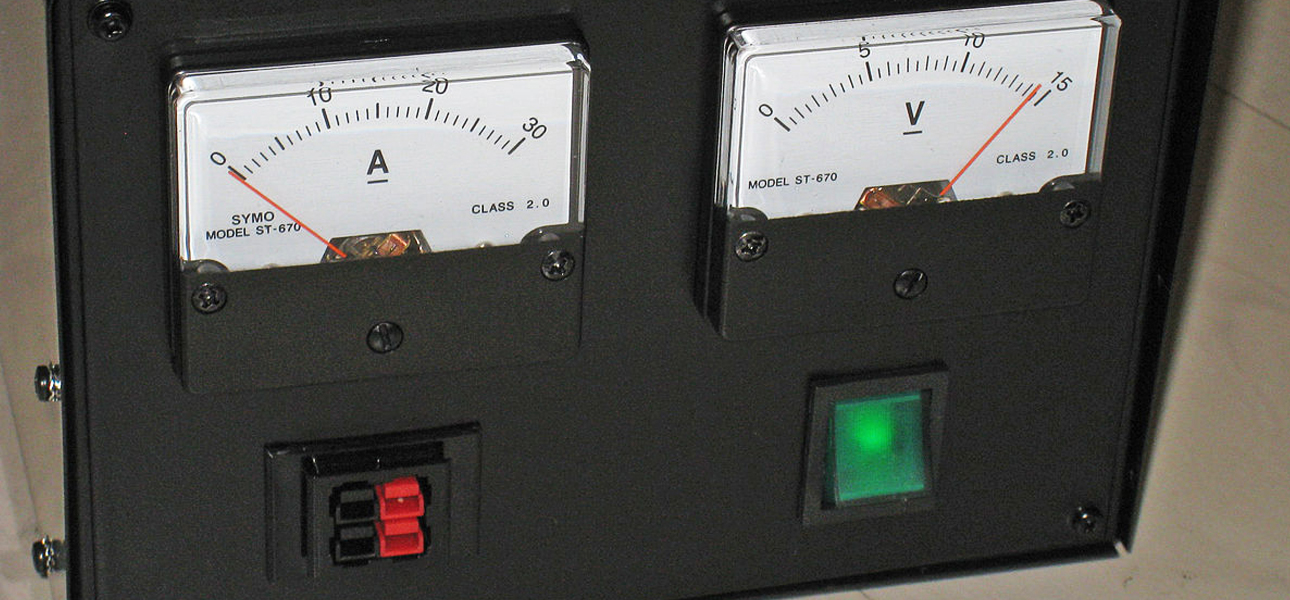# Measuring Units – An OverviewElectricity in its simple terms is the flow of electrons or charges. Electric current flows through substances such as the copper wires or cords you have in your electrical installations. These are also called conductors. A current carrying conductor is said to be energized or live. In many electronic types of equipment electrical current have ratings and units that are used to determine some specifications in connectivity requirements.

The basic electrical units are measured based on the International metric system of measurement, the SI System.

Calculations are done with one or two of the basic units to arrive at a derived unit of measurement. In some other situations, fractions or multiples of these units are used. Some of the basic units used in electricity are:

## VoltThe electrical unit of voltage is volt. It is defined as the energy in joules that is used when an electric charge in coulomb passes through a circuit. The term high voltage and low voltage are familiar ones when dealing with a power surge.

Voltage is represented mathematically for a unit quantity as:

1 V = 1J/ 1 C

The symbol for volt in most cases is V.

The Unit of Electrical Potential is the product of the current, I, passing through a circuit and the resistance, R that opposes current flow in the circuit.

That is, V= I x R

A major higher multiple of voltage is the Kilovolt (KV). That is, 1V x 1000.

A lower fraction also exists such as the millivolt. That is 1V x 0.001.

## AmpereThe unit of electrical current is ampere. It is a measure of the electrical charge that passes through a circuit in one second. The symbol of the ampere is A while current is represented as I.

1A = 1C/1 s.

The unit of electrical current is also measured as:

I = V/R

A lot has been discussed on the need for electrical safety and protection when dealing with electricity.

## WattPower is a major unit in electricity. It is required for you to know the power output or input amount in a device. The electrical unit of power is Watt (W). It is the rate of energy consumed in an electrical circuit.

1W = 1J/1s

1 Kilowatt = 1000 W.

Another derivation of the unit of power is:

P = V x I

P = I2 x R

Decibel-watt (dBW) is a common unit used in some electrical appliances. It uses a logarithmic scale in its measurement.

## OhmsThe unit of resistance is Ohms. Resistance in an electrical circuit is a measure of the degree of the opposition of the resistance to the passage of electricity in the circuit.

Resistance evaluation in a DC electric current is given as:

R = V/I

You can also have multiples or fractions of the unit such as kilo and others.

Many electrical units can be found on most electrical safety devices. Though advanced knowledge about them may not be absolutely necessary, having basic knowledge of the major ones will be adequate